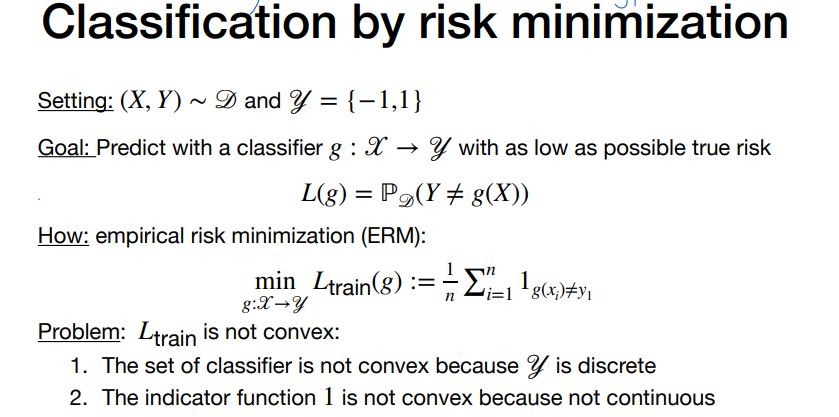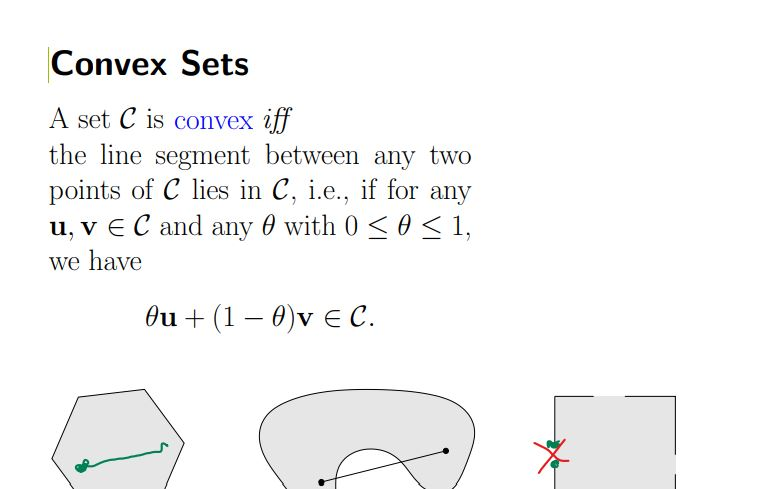### Classification by risk minimization

Hey, I have some questions regarding the following slide:

1) Ltrain is not convex as a function of which variable? as function of classifiers (g)?
2) in the first point, "The set of classifier is not convex because is discrete", convexity here is regarding the definition of convex sets and not convex function right? ( I attached the definition of it in the second picture). If it is about convexity in sets, which set are we talking about here? the set of all classifiers from X to Y ?Top comment

When the question says "L_train" is not convex, this is meant to be "over the space of functions g".

For this to be possible, the "space of functions g" needs to be a convex set. For linear functions, you can easily define 'addition' of the functions (by adding the parameters), and any combination of linear functions would also be a linear function.

For functions that output either 1 or 0, you cannot average those models within the set. An average of a function that outputs 1 and one that outputs 0 should intuitively output 1/2, which is allowed in the set.

Hope this helps.

Page 1 of 1